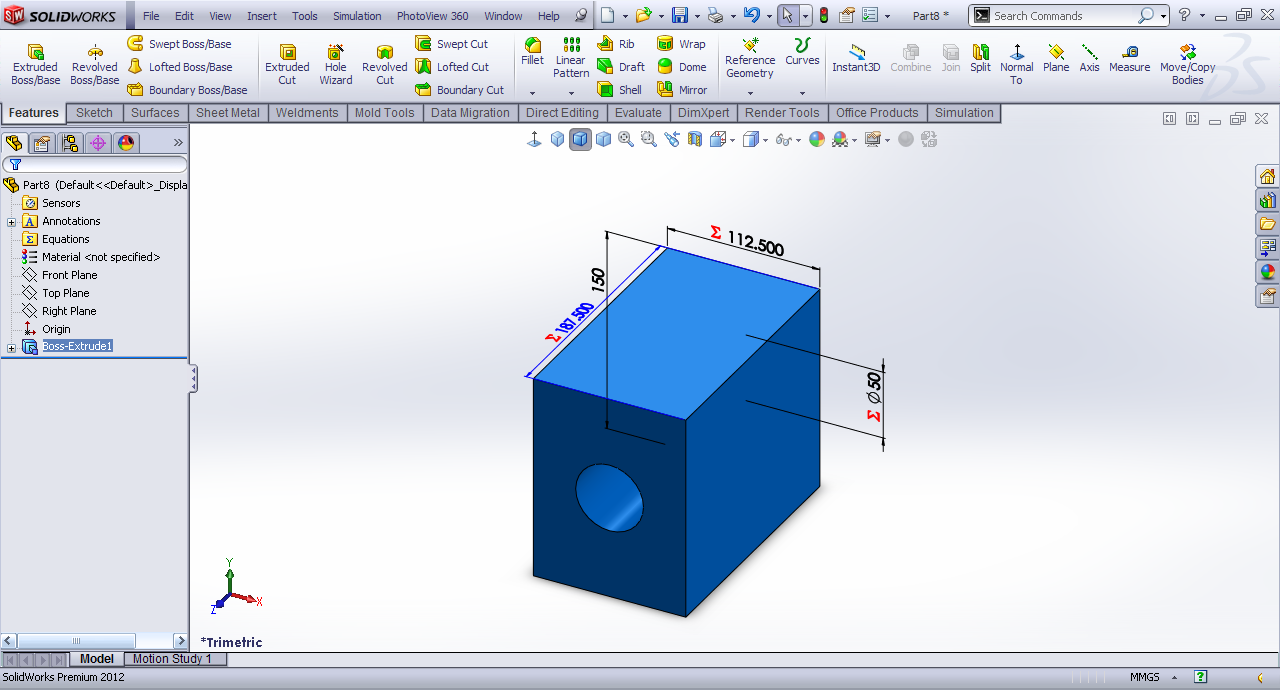# Tutorial - Equation driven part in SolidWorks?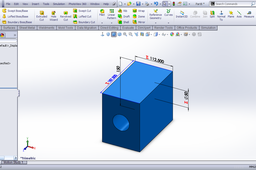Here is the tutorial.

1. ### Step 1:

Front Plane>>Sketch.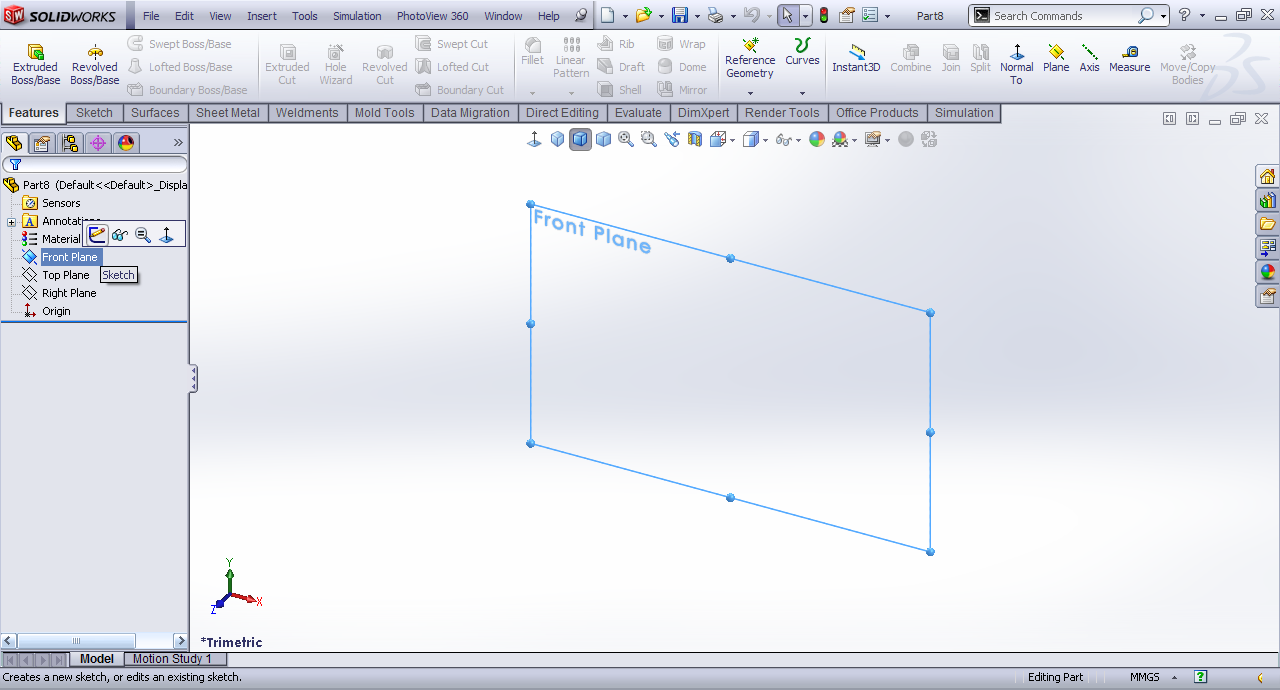2. ### Step 2:

Draw a center point rectangle at origin. Smart Dimension one side.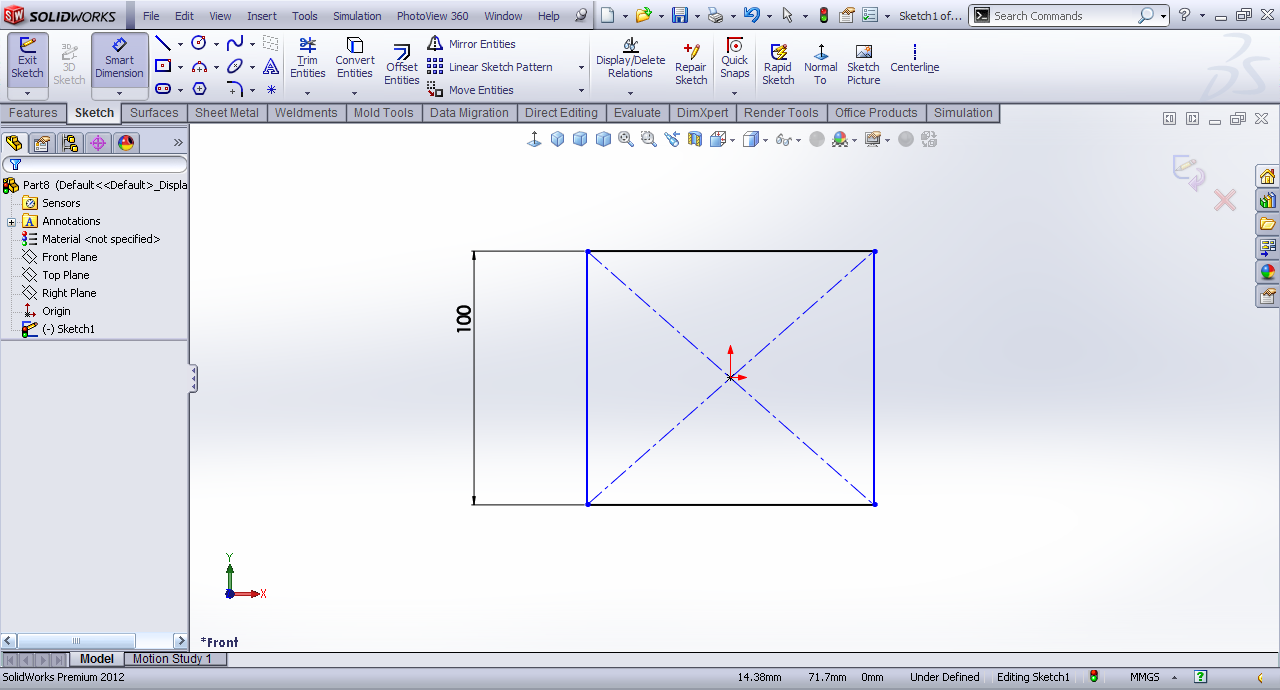3. ### Step 3:

Smart Dimension other side and in value input press =.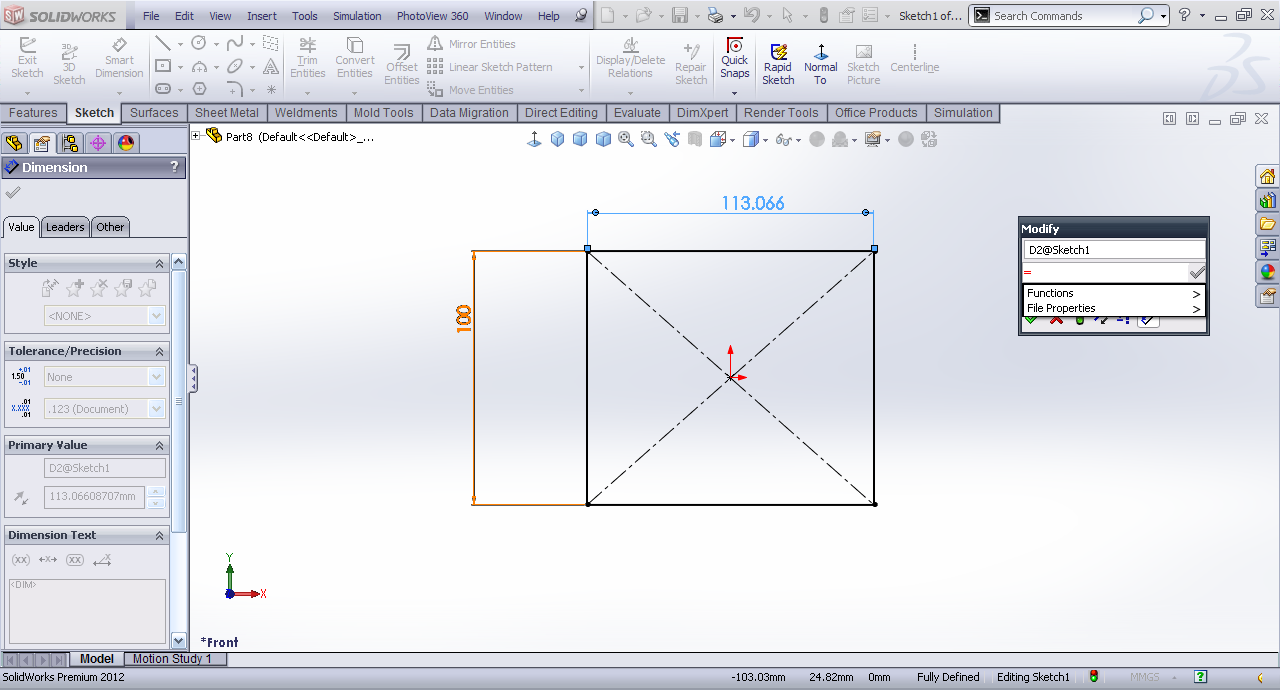4. ### Step 4:

Select the 1st dimension and then *0.75. This will be expression y=0.75*x.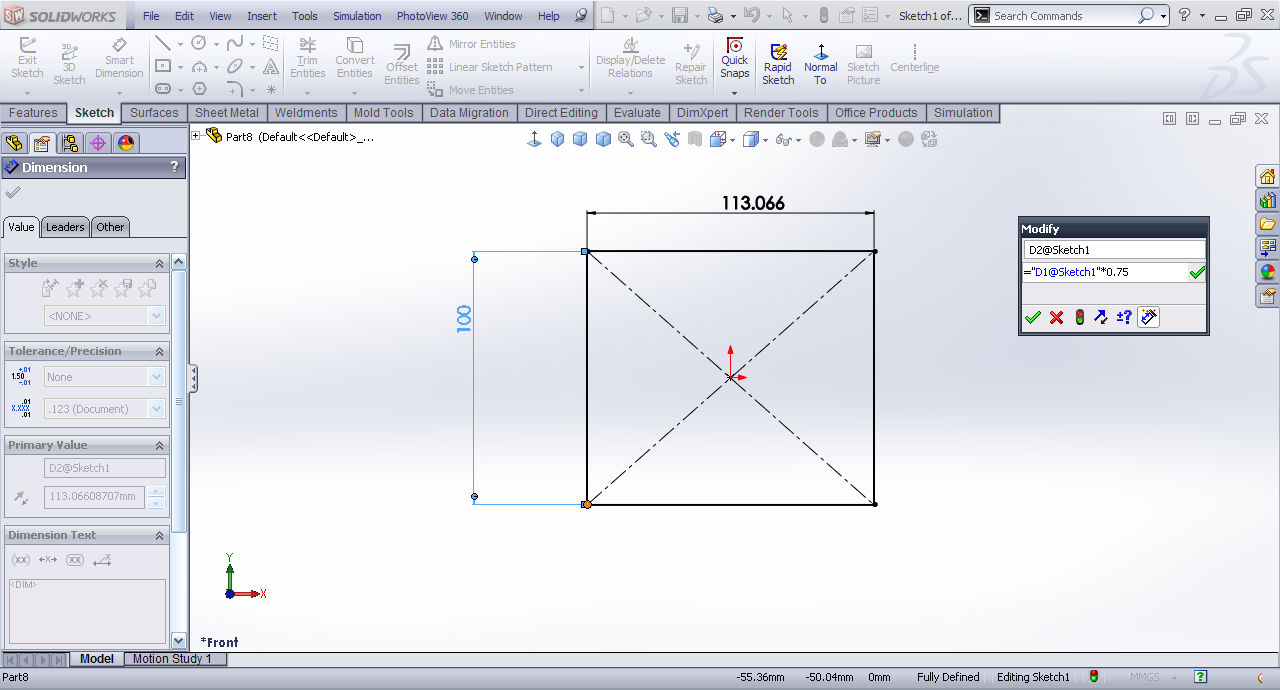5. ### Step 5:

Draw a circle at origin and smart dimension by the first dimension /3. i.e.z=x/3.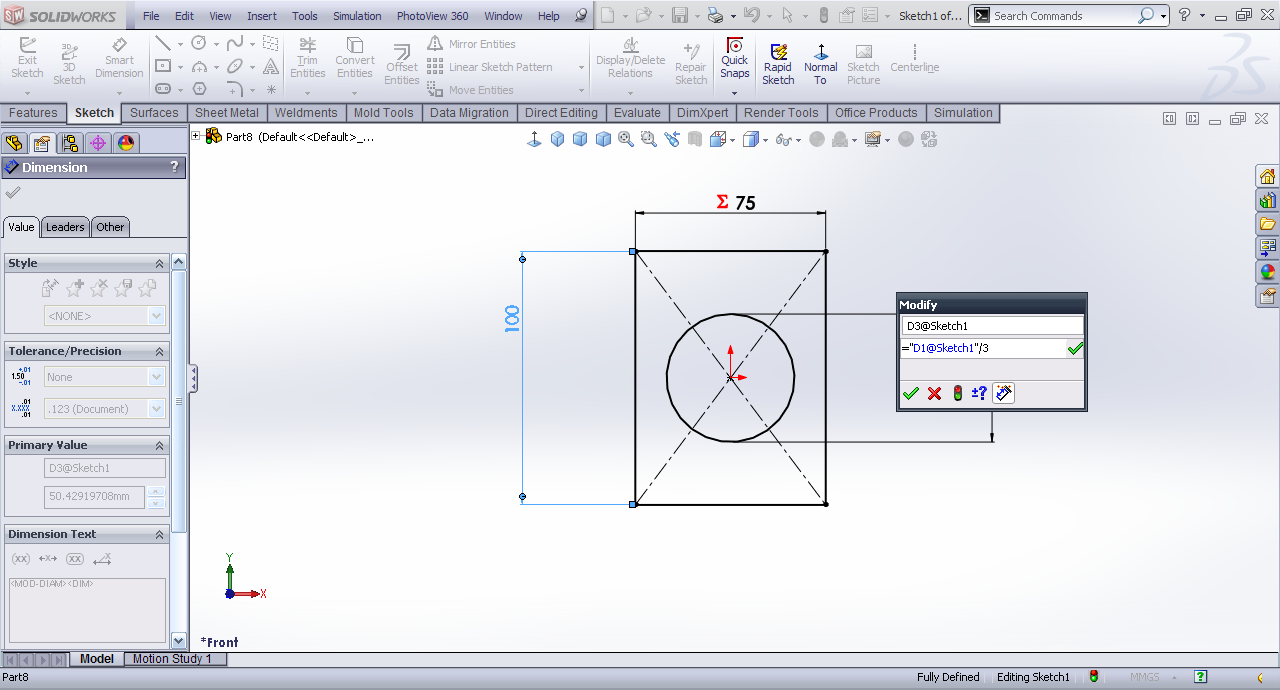6. ### Step 6:

Extrude it.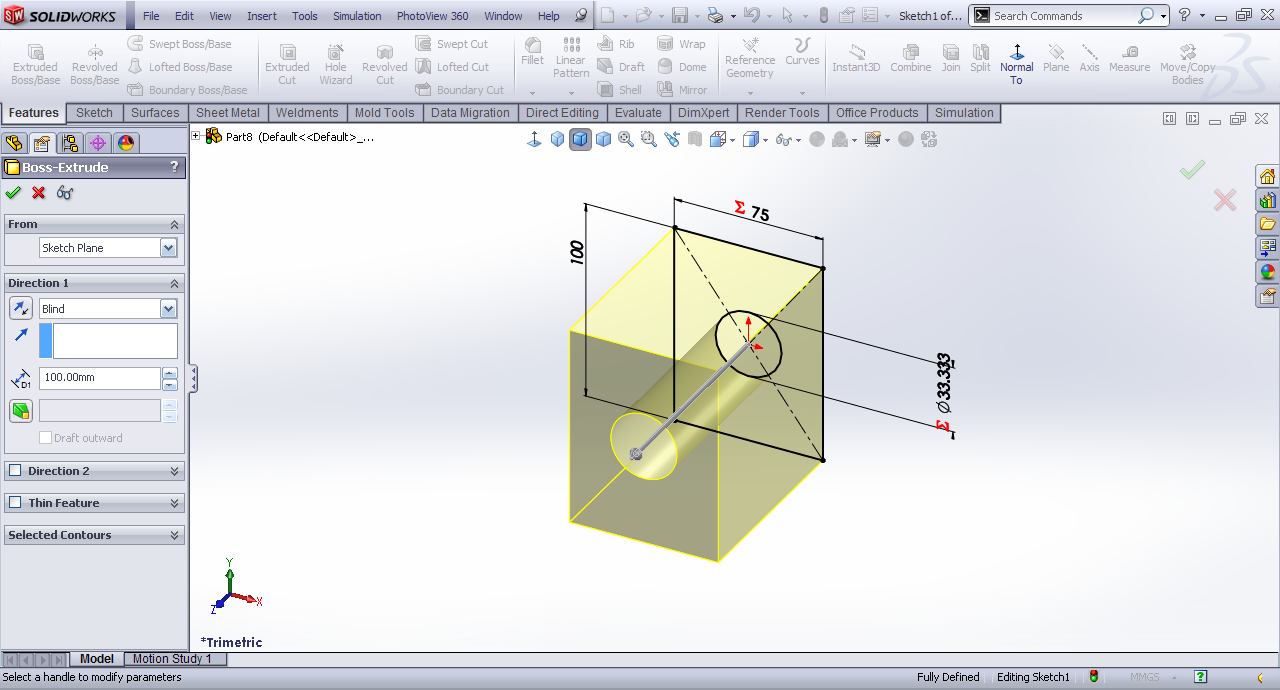7. ### Step 7:

Double click the part. It will show all the dimensions.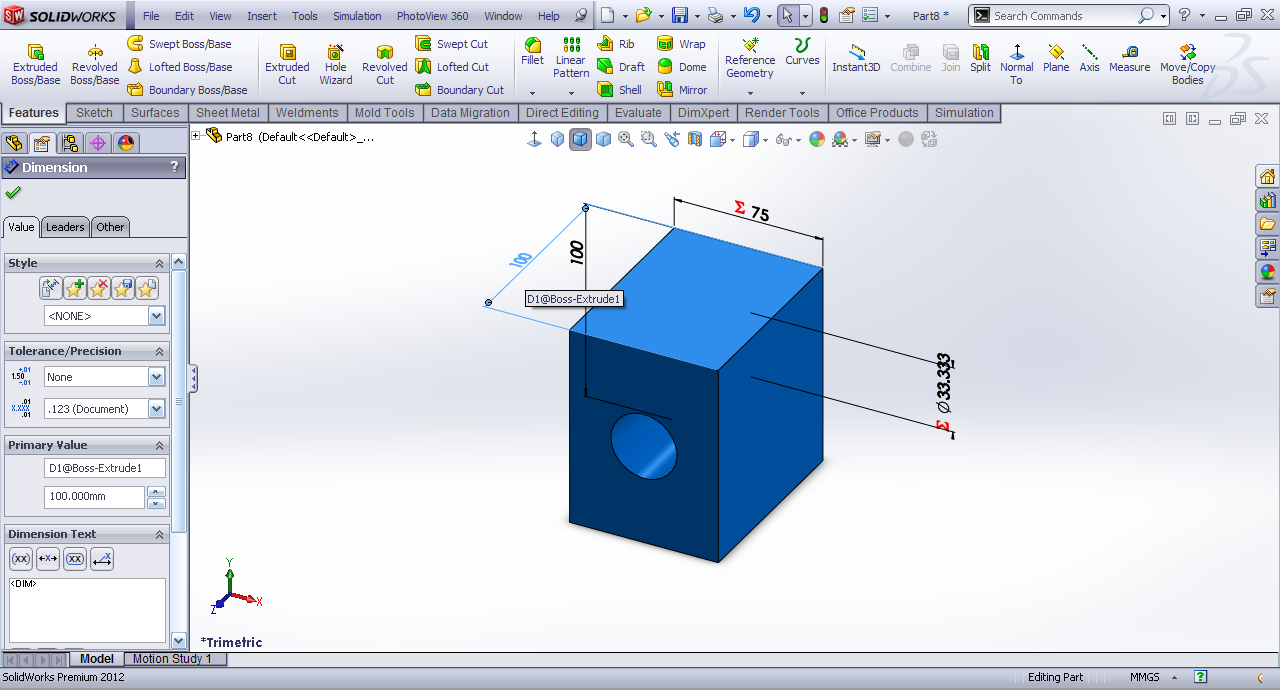8. ### Step 8:

Double click the extrusion dimension and it's relation with the height of part also *1.25. i.e. a=1.25*x.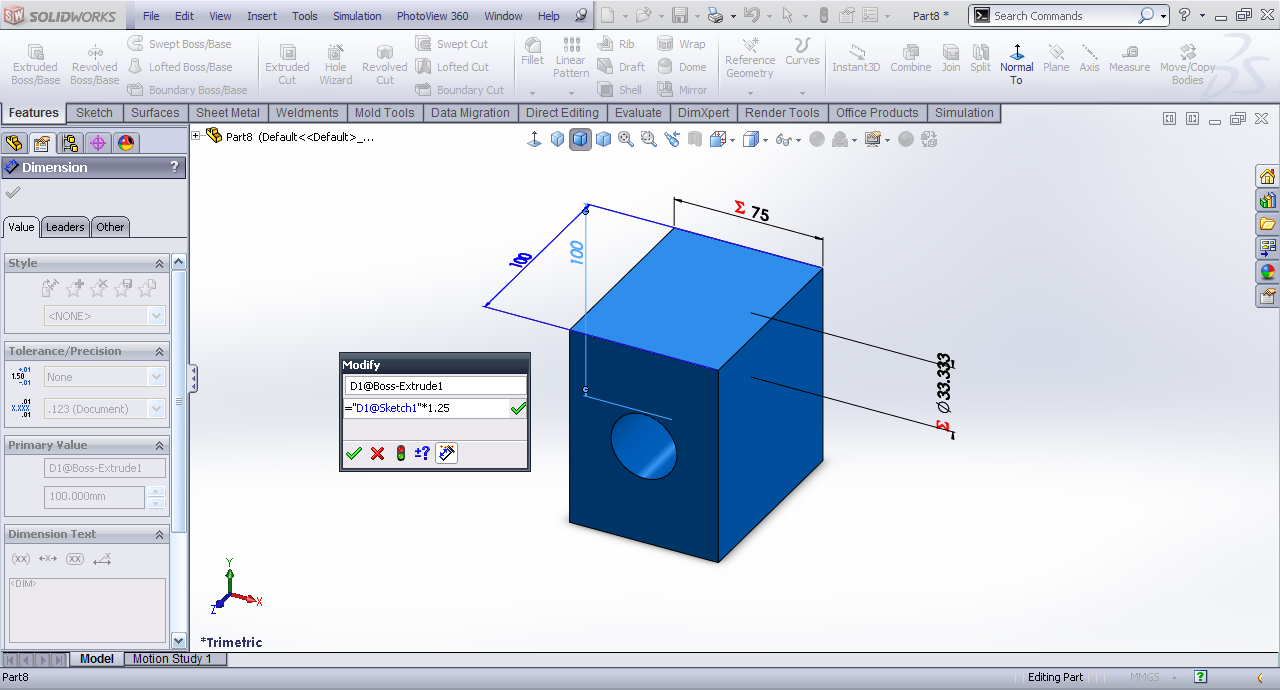9. ### Step 9:

Rebuild part.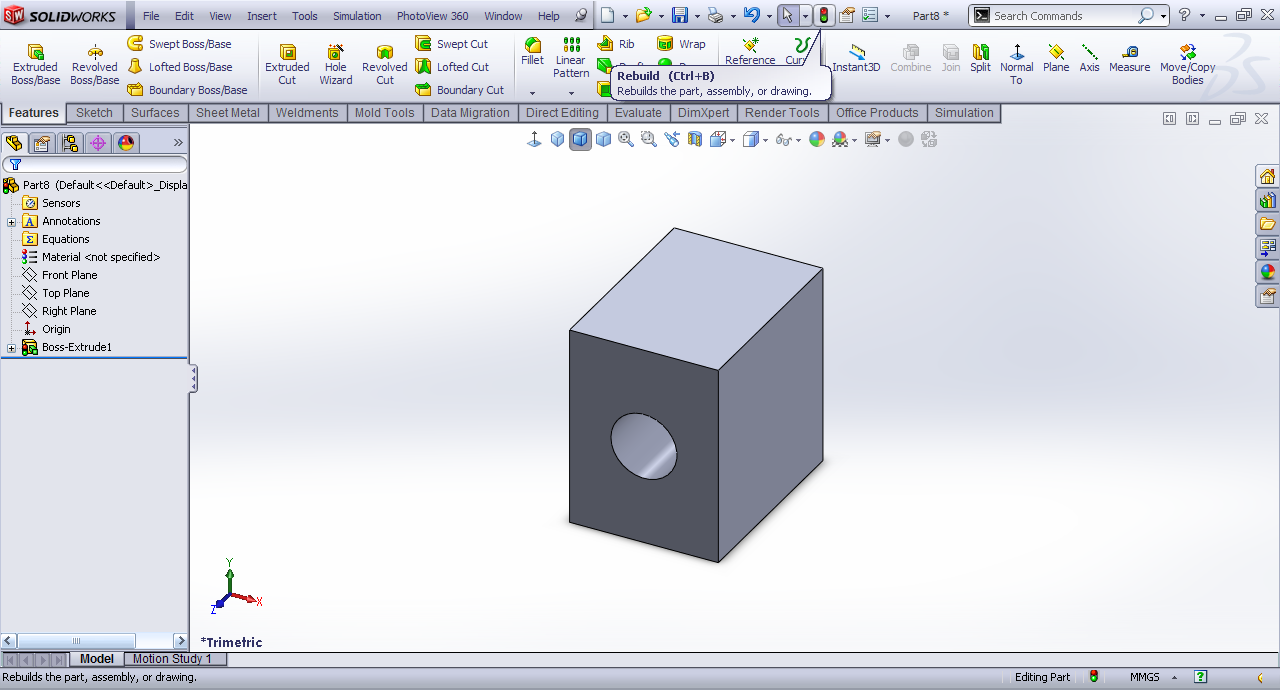10. ### Step 10:

When we change the height of part from 100 to 150.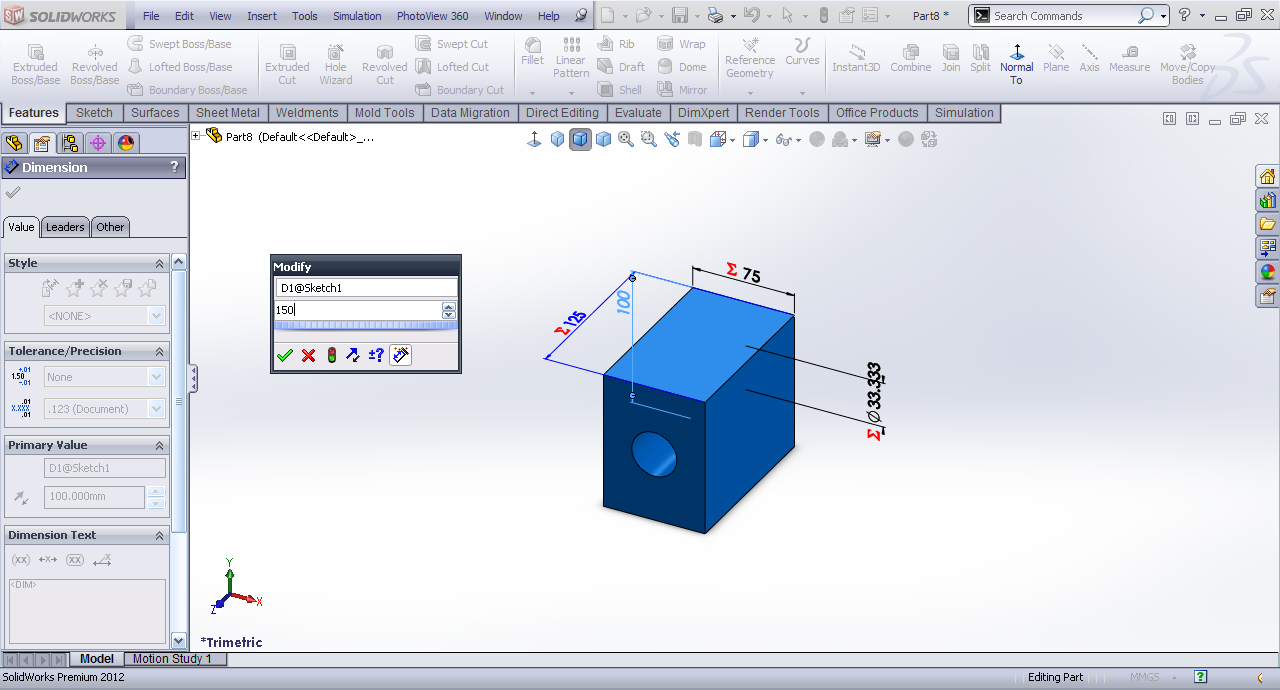11. ### Step 11:

Click Rebuild and we have new dimensions.